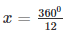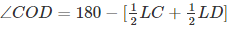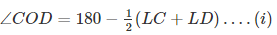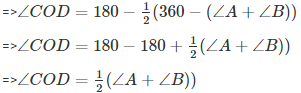Courses

# RD Sharma Solutions Ex-14.1, Quadrilaterals, Class 9, Maths Class 9 Notes | EduRev

## Class 9 : RD Sharma Solutions Ex-14.1, Quadrilaterals, Class 9, Maths Class 9 Notes | EduRev

The document RD Sharma Solutions Ex-14.1, Quadrilaterals, Class 9, Maths Class 9 Notes | EduRev is a part of the Class 9 Course RD Sharma Solutions for Class 9 Mathematics.
All you need of Class 9 at this link: Class 9

1) Three angles of a quadrilateral are respectively equal to 1100, 500 and 400. Find its fourth angle.

Solution:

Given,

Three angles are 1100, 500 and 400

Let the fourth angle be ‘x’

We have,

Sum of all angles of a quadrilateral = 3600

1100 + 500 + 400 = 3600

⇒ x = 3600 – 2000

⇒x = 1600

Therefore, the required fourth angle is 1600.

2) In a quadrilateral ABCD, the angles A, B, C and D are in the ratio of 1:2:4:5. Find the measure of each angles of the quadrilateral.

Solution:

Let the angles of the quadrilaterals be

A = x, B = 2x, C = 4x and D = 5x

Then,

A + B + C + D = 3600

⇒ x + 2x + 4x + 5x = 3600

⇒ 12x = 3600

⇒⇒ x = 300

Therefore, A = x = 300

B = 2x = 600

C = 4x = 1200

D = 5x = 1500

3) In a quadrilateral ABCD, CO and Do are the bisectors of ∠Cand∠D respectively. Prove that ∠COD = 1/2(∠Aand∠B).

Solution:

InΔDOC

∠1+∠COD+∠2=1800          [Angle sum property of a triangle]

⇒ ∠COD = 180−(∠1−∠2)

⇒ ∠COD = 180−∠1+∠2

⇒[∵ OC and Od are bisectors of LC and LD respectively]

⇒∠A+∠B+∠C+∠D = 3600  [Angle sum propert yof quadrilateral]

∠C+∠D=3600−(∠A+∠B)….(ii)

Substituting (ii) in (i)4) The angles of a quadrilateral are in the ratio 3:5:9:13. Find all the angles of the quadrilateral.

Solution:

Let the common ratio between the angles is ‘t’

So the angles will be 3t, 5t, 9t and 13t respectively.

Since the sum of all interior angles of a quadrilateral is 3600

Therefore, 3t + 5t + 9t + 13t = 3600

⇒ 30t = 3600

⇒ t = 120

Hence, the angles are

3t = 3*12 = 360

5t = 5*12 = 600

9t = 9*12 = 1080

13t = 13*12 = 1560

Offer running on EduRev: Apply code STAYHOME200 to get INR 200 off on our premium plan EduRev Infinity!

91 docs

,

,

,

,

,

,

,

,

,

,

,

,

,

,

,

,

,

,

,

,

,

,

,

,

,

,

,

,

,

,

;